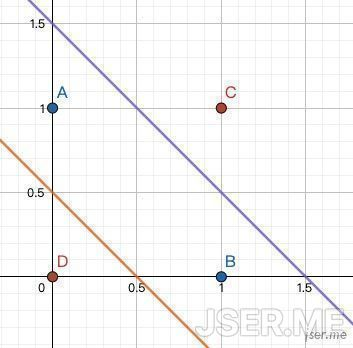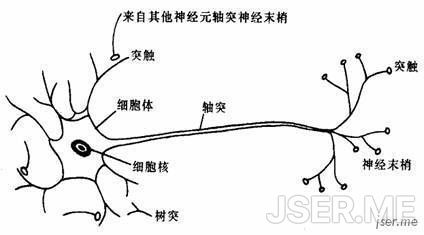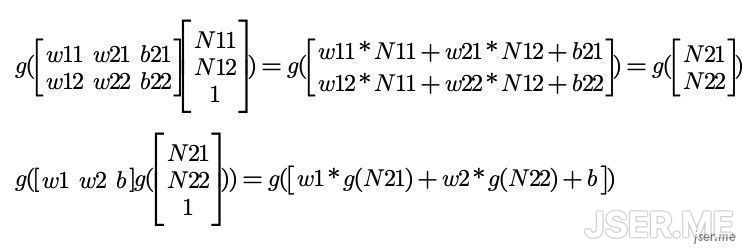# 草依山的Javascript世界# 深度神经网络入门

## 简单预测器

``````\$=w￥
``````

w 误差 w增加值
5 2.15 null
10 -2.85 5
6 1.15 -4
7 0.15 1
7.1 0.05 0.1
7.2 -0.05 0.1
7.15 0 -0.05A 2.61 4.46
S 4.47 2.46 绿``````1 ^ 0 = 1
0 ^ 1 = 1
0 ^ 0 = 0
1 ^ 1 = 0
``````## 神经元（感知机）## 神经网络(多层感知机)### 反向传播算法`1^0=1`，目标的数据应该是1，在简单预测器里我们使用的简单的差来评估误差，这里我们来使用差的平方`1/2(目标值-计算值)^2`(1/2是为了后面计算方便），这个评估误差的函数也叫损失函数或者代价函数(loss function)，训练调整w的目标是使用误差最小，也就是误差函数的值趋于0，从前面的计算可以看出，所有的 w 都和误差相关，那我们应该怎么找到使误差最小的每一个 w 的值呢？``````const tf = require('@tensorflow/tfjs-node')

const model = tf.sequential()
model.add(tf.layers.dense({ inputShape: , units: 2, activation: 'sigmoid' }))
model.add(tf.layers.dense({ units: 1, activation: "sigmoid" }))

model.compile({ optimizer: tf.train.sgd(0.5), loss: 'meanSquaredError' });

const data = tf.tensor([[0, 0], [0, 1],[1, 1], [1, 0]])
const target = tf.tensor([0, 1, 0, 1], [4, 1]);

(async function () {
await model.fit(data, target, {
batchSize: 3,
epochs: 3000,
callbacks: tf.node.tensorBoard('./fit_logs_1')
})

const predict1 = await model.predict(tf.tensor([0, 0], [1, 2])).data()
console.log(`0, 0 : \${predict1}`)

const predict2 = await model.predict(tf.tensor([1, 0], [1, 2])).data()
console.log(`1, 0 : \${predict2}`)
})()
/*

0, 0 : 0.04225059226155281
1, 0 : 0.9512262940406799
*/
``````

## 卷积神经网络``````const tf = require('@tensorflow/tfjs');

const model = tf.sequential();
inputShape: [28, 28, 1],
filters: 32,
kernelSize: 3,
activation: 'relu',
}));
filters: 32,
kernelSize: 3,
activation: 'relu',
}));
filters: 64,
kernelSize: 3,
activation: 'relu',
}));
filters: 64,
kernelSize: 3,
activation: 'relu',
}));

model.compile({
optimizer: 'rmsprop',
loss: 'categoricalCrossentropy',
metrics: ['accuracy'],
});

module.exports = model;
``````

## 总结

Github上也有一些东西：[Github]

2019-09-30

## 相关文章

2017-03-22 一次算PI的小尝试
2017-02-13 new做了些什么
2016-09-29 [翻译]bash的各种文件载入执行顺序
2016-05-31 phantomjs在linux下截图中文字体问题
2016-04-24 Promise的错误处理